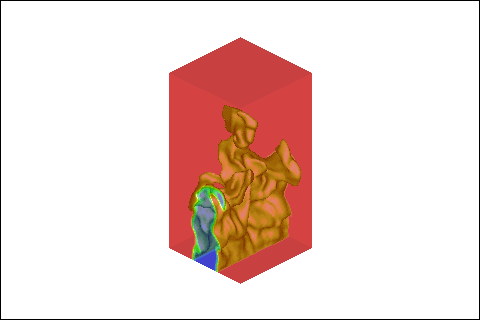Heat, Flow, Combustion, Problems, Analysis, Solutions, Help, Bastiaans### <!-- Begin var day=""; var month=""; var ampm=""; var ampmhour=""; var myweekday=""; var weekday=""; var year=""; mydate = new Date(); myday = mydate.getDay(); mymonth = mydate.getMonth(); myweekday= mydate.getDate(); weekday= myweekday; myyear= mydate.getYear(); year = myyear; myhours = mydate.getHours(); ampmhour = (myhours > 12) ? myhours - 12 : myhours; ampm = (myhours >= 12) ? ' PM' : ' AM'; mytime = mydate.getMinutes(); myminutes = ((mytime < 10) ? ':0' : ':') + mytime; if(myday == 0) day = " Sunday, "; else if(myday == 1) day = " Monday, "; else if(myday == 2) day = " Tuesday, "; else if(myday == 3) day = " Wednesday, "; else if(myday == 4) day = " Thursday, "; else if(myday == 5) day = " Friday, "; else if(myday == 6) day = " Saturday, "; if(myweekday == 1) weekday = "1st "; else if(myweekday == 21) weekday = "21st "; else if(myweekday == 2) weekday = "2nd "; else if(myweekday == 22) weekday = "22nd "; else if(myweekday == 23) weekday = "23rd "; else if(myweekday == 3) weekday = "3rd "; else if(myweekday == 31) weekday = "31st "; else weekday = myweekday + "th "; if(mymonth == 0) {month = "January";} else if(mymonth ==1) month = "February"; else if(mymonth ==2) month = "March"; else if(mymonth ==3) month = "April"; else if(mymonth ==4) month = "May"; else if(mymonth ==5) month = "June"; else if(mymonth ==6) month = "July"; else if(mymonth ==7) month = "August"; else if(mymonth ==8) month = "September"; else if(mymonth ==9) month = "October"; else if(mymonth ==10) month = "November"; else if(mymonth ==11) month = "December"; r = myyear - 100; if (myyear >= 1999) year = " " + myyear; else if(r < 10) year = " 200" + r; else year = " 20" + r; //--># News & events

## Events Calendar

#### 25th "Journées D'étude", May 15th to 16th, 2018, Mons, Belgium. Combustion-DNS Strategy & Data Analyses workshop, May 22nd to 23th, 2018, Sorrento, Italy. Turbo Expo, June 11th to 15th, 2018, Oslo, Norway. 37th International Symposium on Combustion, 29 July to 3 August 2018, Dublin, Ireland. Combura 2018, 10 October 2018, Soesterberg, The Netherlands.